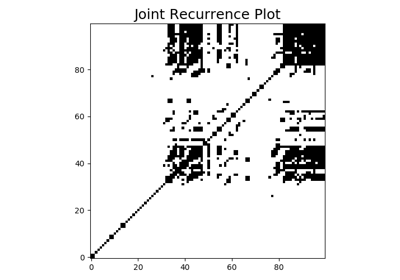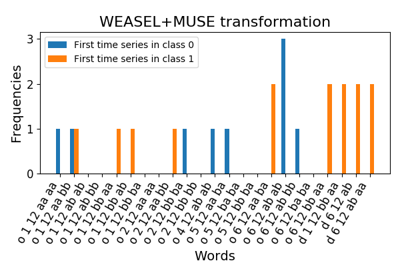# `pyts.datasets`.load_basic_motions¶

`pyts.datasets.``load_basic_motions`(return_X_y=False)[source]

Load and return the Basic Motions dataset.

The data was generated as part of a student project where four students performed four activities whilst wearing a smart watch. The watch collects 3D accelerometer and a 3D gyroscope It consists of four classes, which are walking, resting, running and badminton. Participants were required to record motion a total of five times, and the data is sampled once every tenth of a second, for a ten second period.

 Training samples 40 Test samples 40 Dimensionality 6 Timestamps 100 Classes 4
Parameters: return_X_y : bool (default = False) If True, return `(data_train, data_test, target_train, target_test)` instead of a Bunch object. data : Bunch Dictionary-like object, with attributes: data_train : array of floats The time series in the training set. data_test : array of floats The time series in the test set. target_train : array of integers The classification labels in the training set. target_test : array of integers The classification labels in the test set. DESCR : str The full description of the dataset. url : str The url of the dataset. (data_train, data_test, target_train, target_test) : tuple if `return_X_y` is True

References

Examples

```>>> from pyts.datasets import load_basic_motions
>>> bunch.data_train.shape
(40, 6, 100)
>>> X_train, X_test, y_train, y_test = load_basic_motions(return_X_y=True)
>>> X_train.shape
(40, 6, 100)
```

## Examples using `pyts.datasets.load_basic_motions`¶Joint Recurrence PlotWEASEL+MUSE